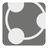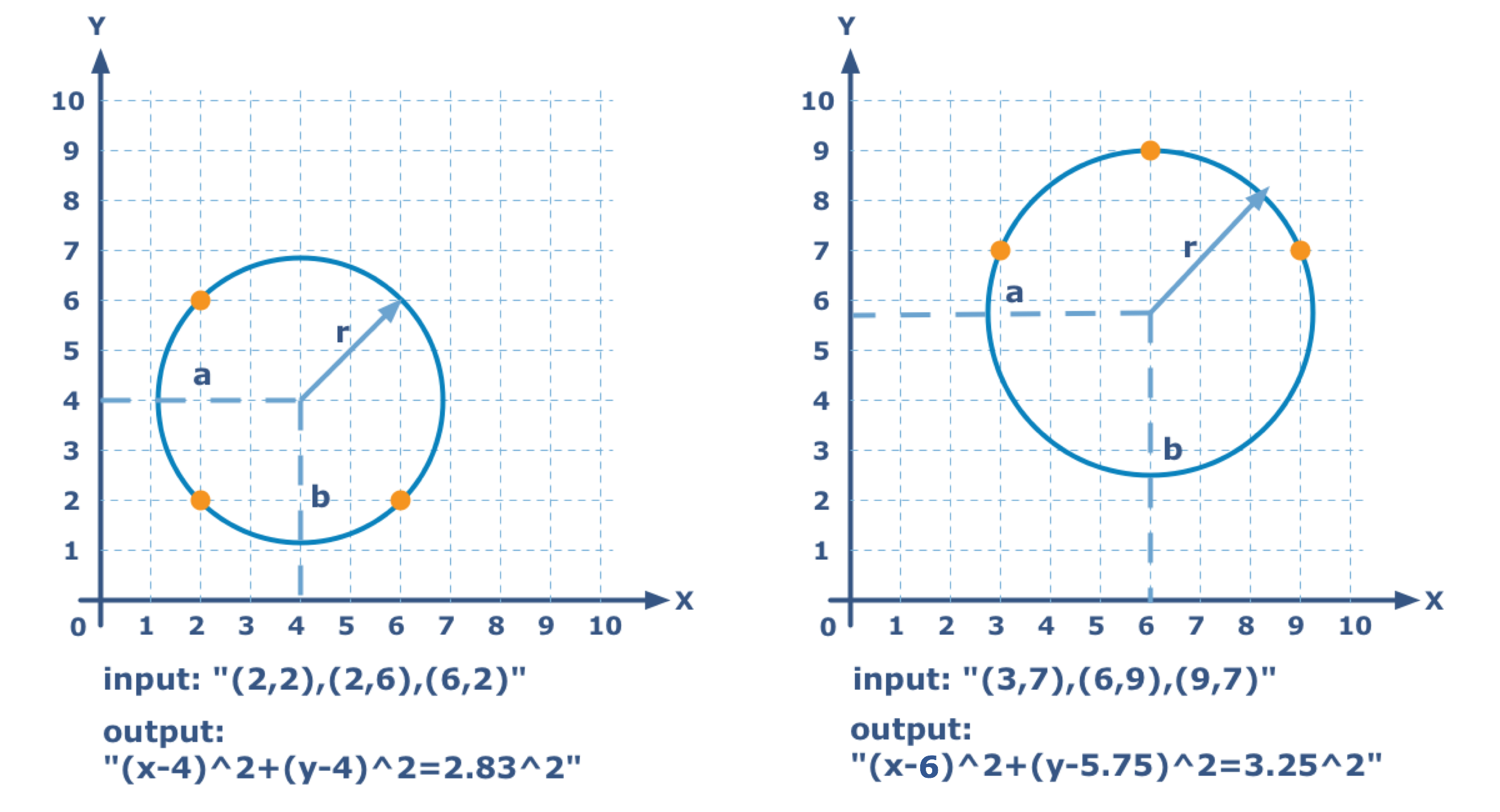Three Points Circle
Simple
English FR RU

Nicola discovered a caliper inside a set of drafting tools he received as a gift. Seeing the caliper, he has decided to learn how to use it.

Through any three points that do not exist on the same line, there lies a unique circle. The points of this circle are represented in a string with the coordinates like so:

"(x1,y1),(x2,y2),(x3,y3)"

Where x1,y1,x2,y2,x3,y3 are digits.

You should find the circle for three given points, such that the circle lies through these point and return the result as a string with the equation of the circle. In a Cartesian coordinate system (with an X and Y axis), the circle with central coordinates of (x0,y0) and radius of r can be described with the following equation:

"(x-x0)^2+(y-y0)^2=r^2"

where x0 , y0 , r are decimal numbers rounded to two decimal points . Remove extraneous zeros and all decimal points, they are not necessary. For rounding, use the standard mathematical rules.Input: Coordinates as a string..

Output: The equation of the circle as a string.

Example:

checkio("(2,2),(6,2),(2,6)") == "(x-4)^2+(y-4)^2=2.83^2"
checkio("(3,7),(6,9),(9,7)") == "(x-6)^2+(y-5.75)^2=3.25^2"

How it is used: This equation, also known as Equation of the Circle, comes from the Pythagorean theorem when applied to any point on a circle: the radius is the hypotenuse of a right-angled triangle whose other sides are of length x − a and y − b. Of course you can use this concept for you mathematics software, but we just want to remind about how awesome circles are.

Precondition: All three given points do not lie on one line.
0 < x i , y i , r < 10

Settings
Code:
CheckiO Extensions

CheckiO Extensions allow you to use local files to solve missions. More info in a blog post.

In order to install CheckiO client you'll need installed Python (version at least 3.8)

Install CheckiO Client first:

pip3 install checkio_client

checkio --domain=py config --key=

Sync solutions into your local folder

checkio sync

(in beta testing) Launch local server so your browser can use it and sync solution between local file end extension on the fly. (doesn't work for safari)

checkio serv -d

Alternatevly, you can install Chrome extension or FF addon

checkio install-plugin
checkio install-plugin --ff
checkio install-plugin --chromium

Read more here about other functionality that the checkio client provides. Feel free to submit an issue in case of any difficulties.

Sync...